## Table of ContentOpen Access

ARTICLE

# The Class of Atomic Exponential Basis Functions EFupn(x,ω)-Development and Application

Nives Brajčić Kurbaša*, Blaž Gotovac, Vedrana Kozulić

University of Split, Faculty of Civil Engineering, Architecture and Geodesy, Split, 21000, Croatia

* Corresponding Author:Nives Brajčić Kurbaša. Email:Computer Modeling in Engineering & Sciences 2023, 135(1), 65-90. https://doi.org/10.32604/cmes.2022.021940

## Abstract

The purpose of this paper is to present the class of atomic basis functions (ABFs) which are of exponential type and are denoted by . While ABFs of the algebraic type are already represented in the numerical modeling of various problems in mathematical physics and computational mechanics, ABFs of the exponential type have not yet been sufficiently researched. These functions, unlike the ABFs of the algebraic type , contain the tension parameter , which gives them additional approximation properties. Exponential monomials up to the th degree can be described exactly by the linear combination of the functions . The function for is called the “mother” ABF of the exponential type, i.e., . In other words, the functions are elements of the linear vector space and retain all the properties of their “mother” function . Thus, this paper, in terms of its content and purpose, can be understood as a sequel of the article by Brajčić Kurbaša et al., which shows the basic properties and application of the basis function This paper presents, in an analogous way, the development and application of the exponential basis functions . Here, for the first time, expressions for calculating the values of the functions and their derivatives are given in a form suitable for application in numerical analyses, which is shown in the verification examples of the approximations of known functions.

## Keywords

1  Introduction

Numerical methods are indispensable for the successful simulation of physical and engineering problems. Many different numerical approaches and methods have been proposed in recent decades. The classical methods are the finite element method (FEM), the finite difference method (FDM), the finite volume method (FVM), the boundary element method (BEM), and the discrete element method (DEM) . In addition to traditional mesh-based methods, there are many others, such as various meshless methods .

The choice of the basis functions plays a key role in all numerical methods. The idea of choosing basis functions that correspond to the class of solutions of the problems we are solving has long been accepted, but, in practice, rarely implemented. Polynomials are fundamental to modeling and numerical methods. They provide canonical local approximations to smooth functions and are used extensively in geometric design. Polynomials not only provide very accurate approximations of smooth functions but also guarantee convergence for any continuous function on a compact interval.

Whereas classical polynomials have dominated in the field of numerical analysis, spline-based basis functions  play a crucial role in the field of computational geometry. The true popularity of spline functions for numerical analysis was achieved by the introduction of the concept of isogeometric analysis (Hughes et al.  and Cottrell et al. ). B-splines play an important role in many areas of applied mathematics, computer science, and engineering. Typical applications arise in the approximation of functions and data, automated design and manufacturing, computer graphics, and numerical simulations. This diversity of areas and techniques involved makes B-splines an extremely interesting research topic, which has attracted a growing number of scientists in universities and industry.

In addition to spline functions, relatively lesser-known atomic basis functions have been used in recent times . Atomic basis functions can be placed between classical polynomials and spline functions. However, in practice, their use as basis functions is closer to splines or wavelets (see Beylkin et al. ). Rvachev et al. , in their pioneering work, called these basis functions “atomic” because they span the vector spaces of all three fundamental functions in mathematics: algebraic, exponential, and trigonometric polynomials. The authors of this article have worked intensively on the development and application of ABFs of algebraic type in solving problems of structural mechanics and have therefore demonstrated their significant potential compared to conventional procedures with finite elements. Gotovac  systematized the existing knowledge regarding atomic basis functions of algebraic type and transformed them into a numerically appropriate form, especially Fup basis functions as a typical member of the atomic class of basis functions. Gotovac et al.  showed the basic possibilities of using atomic functions in structural mechanics and numerical analysis. The work in  gives a generalization of atomic functions to the multivariable case. The use of Fup basis functions, which are atomic functions of the algebraic type, has been shown to solve the problem of signal processing , the initial value problem , the boundary value problems using the Fup Collocation Method , the boundary-initial value problems , elasto-plastic analysis of prismatic bars subjected to torsion , and modeling of groundwater flow and transport problems . Gotovac et al.  presented a true multiresolution approach based on the Adaptive Fup Collocation Method (AFCM). Kamber et al.  set the foundation for an efficient adaptive spatial procedure by developing a one-dimensional hierarchical Fup (HF) basis functions. The works in [25,26] gave a brief analysis of the current publications regarding ABFs, from the first publications to current ones.

In the mentioned works, the advantage of atomic basis functions of algebraic type, which significantly improve the quality of numerical solutions in relation to classical basis functions, for example, splines and wavelets, is confirmed. The numerical results thus obtained were the motivation for the development of ABF of the exponential type which are wider than algebraic space, moreover algebraic ABFs space is contained in exponential ABFs space.

The numerical modeling of different physical and engineering problems characterized by large local gradients and singularities often presents a challenge in terms of choosing a numerical approach and basis functions. Classic examples of such are the advection–dispersion equation and the heat conduction equation, which describe the transfer of mass and energy, respectively; beams and plates on a flexible foundation; and special problems of loss of stability. For the simulation of such physical problems, exponential basis functions would be a good choice. Improving the quality of numerical analyzes of problems whose solutions have an exponential form is the main motivation of this paper.

The atomic functions of the exponential type have been developed only at the basic level. In , the previous knowledge about ABF of the exponential type was presented, which was later expanded and upgraded in . Reference , partly resulted from , showed the basic properties and application of the maternal basis function Eup(x,ω), by which the whole class of atomic functions of the exponential type EFupn(x,ω) is generated and given in this article as natural sequel of the  to complete “the story” of the ABFs of the exponential type.

The content of this work is focused on the mathematical background, approximation properties, and applications of exponential basis functions EFupn(x,ω). There are no articles in the literature that deal with these basis functions. So, this paper is intended to provide novel information for scientists and engineers who are interested in applying the state-of-the-art atomic exponential basis functions to solve real-life problems. The paper presents expressions for the necessary mathematical operations of the ABFs EFupn(x,ω) in a simpler, more understandable and more user-friendly way. New expressions have been derived, especially the expression for calculating the value of the function and the desired number of derivatives at an arbitrary point of the basis function support, which is the original contribution of this paper and, most importantly, the rules (elements) for their practical use.

The following section of the article refers to the description of the ABF class of the algebraic type. The procedure used to generate the class of functions Fupn(x) and the determination of their derivatives are presented, and the basic properties are given in a new and original way, and that is starting from the well-known Fourier transform and the convolution theorem in a way suitable for defining and deriving the ABFs of the exponential type EFupn(x,ω), shown in Section 3. The implementation of ABF EFupn(x,ω) in the numerical approximations of the given functions is shown in Section 4. Finally, the conclusions are given in Section 5.

2  ABFs of the Algebraic Type: Fupn (x)

Atomic Basis Functions (ABFs) are infinitely derivable finite solutions of functional differential equations of the type:

Ly(x)=λk=1MCky(axbk),(1)

where L is a linear differential operator with constant coefficients, λ is a scalar quantity other than zero, Ck are the solution coefficients, a>0 is the support length parameter of the finite function, and bk are the coefficients that determine the displacements of the finite basis functions [1012,15].

The type of finite function y(x) from the class of atomic basis functions is determined by choosing the operator L in Eq. (1). Thus, we distinguish the atomic basis functions of the algebraic, exponential, and trigonometric types.

The functions Fupn(x) are finite ABFs of the algebraic type from the class C with a compact support, and they are also elements of the universal vector space UPn. The index n denotes the highest degree of a polynomial that can be accurately represented in the form of a linear combination of basis functions obtained by moving the function Fupn(x) for the characteristic segment Δxn=2n. For n=0, it holds that:

Fup0(x)up(x).(2)

The functions Fupn(x) retain all the good properties of the “maternal” function up(x) [1012,15], while for the development of a given function, a much smaller number than that of the basis functions obtained by moving the function up(x) is required. For a sufficiently high n function Fupn(x) has a very small support length, so any function Fupk(x),k<n, including the function up(x), can be expressed using the function Fupn(x).

Unlike in references [10,11] which define ABFs from Eq. (1), the authors of this paper determine ABFs from their known Fourier transform (FT), and then from their known FT determine everything necessary for their use (e.g., derivatives, integrals, moments, etc.). Namely, we can say that in the “frequency domain” the construction of ABFs becomes more transparent.

The FT of the function Fup0(x), according to Eq. (2), corresponds exactly to the FT of the function up(x) from [15,28], i.e.,

F0(t)=j=1sin(t2j)t2j.(3)

The Fourier transform of the function Fupn(x) is given by the expression [15,28]:

Fn(t)=(sin(t2n1)t2n1)n+1j=n+2sin(t2j)t2j.(4)

Thus, according to Eq. (4), the functions Fupn(x) can be written in integral form:

Fupn(x)=12πeitxFn(t)dt.(5)

From the known FT, as is shown similarly for the function up(x) in , the functions Fupn(x) can also be generated using the convolution theorem. In Eq. (4), it is seen that the FTs Fn(t) of the basis functions Fupn(x) are equal to the product of the nth degree B-spline FT compressed on the support of length (n+1)2n and the function up(x) FT from Eq. (3) compressed on a support of length 2n. Thus, the functions Fupn(x) can be written using the convolution theorem in the form:

Fupn(x)=Bn(2nx)up(2n+1x).(6)

According to Eq. (6), the support of the function Fupn(x) is an interval composed of n+2 segments of length 2n, which are called characteristic segments, that is,

suppFupn(x)=[(n+2)2n1,(n+2)2n1].(7)

The functional differential equations of the basis functions Fupn(x) are of the following form [12,15]:

Fupn(x)=2k=0n+2(CnkCnk2)Fupn(2xk2n+n+22n+1),(8)

where Cnk are binomial coefficients.

Solving the functional differential Eq. (8), or Eqs. (4) and (5), is not numerically convenient for calculating the values of the function Fupn(x). Practically, the most convenient possibility to construct the functions Fupn(x) is in the form of a linear combination of functions up(x) mutually shifted for the characteristic segment 2n, i.e.,

Fupn(x)=k=0Ck(n)up(x1k2n+n+22n+1).(9)

The “zeroth” coefficient follows from Eq. (9) and is

C0=2Cn+12=2n(n+1)/2.(10)

The other coefficients are obtained in the form Ck(n)=C0(n)Ck(n),, where the auxiliary coefficients Ck(n) are calculated by the recursive formula :

C0(n)=1,fork=0;fork>0:Ck(n)=(1)kCn+1kj=1min{k;2n+11}Ckj(n)δj+1.(11)

The coefficients from Eq. (11) for n6 and k9 are given in Table 1.The derivatives of the function Fupn(x) are obtained by a linear combination of the derivatives of the shifted functions up(x) using the coefficients from Eq. (11), i.e.,

Fupn(m)(x)=k=0Ck(n)up(m)(x1k2n+n+22n+1),(12)

where m is the order of derivation, and n is the order of the basis function. Fig. 1 shows the function Fup2(x) and its first three derivatives. The third, and all further derivatives, of the function Fup2(x) correspond in parts to the compressed function up(x).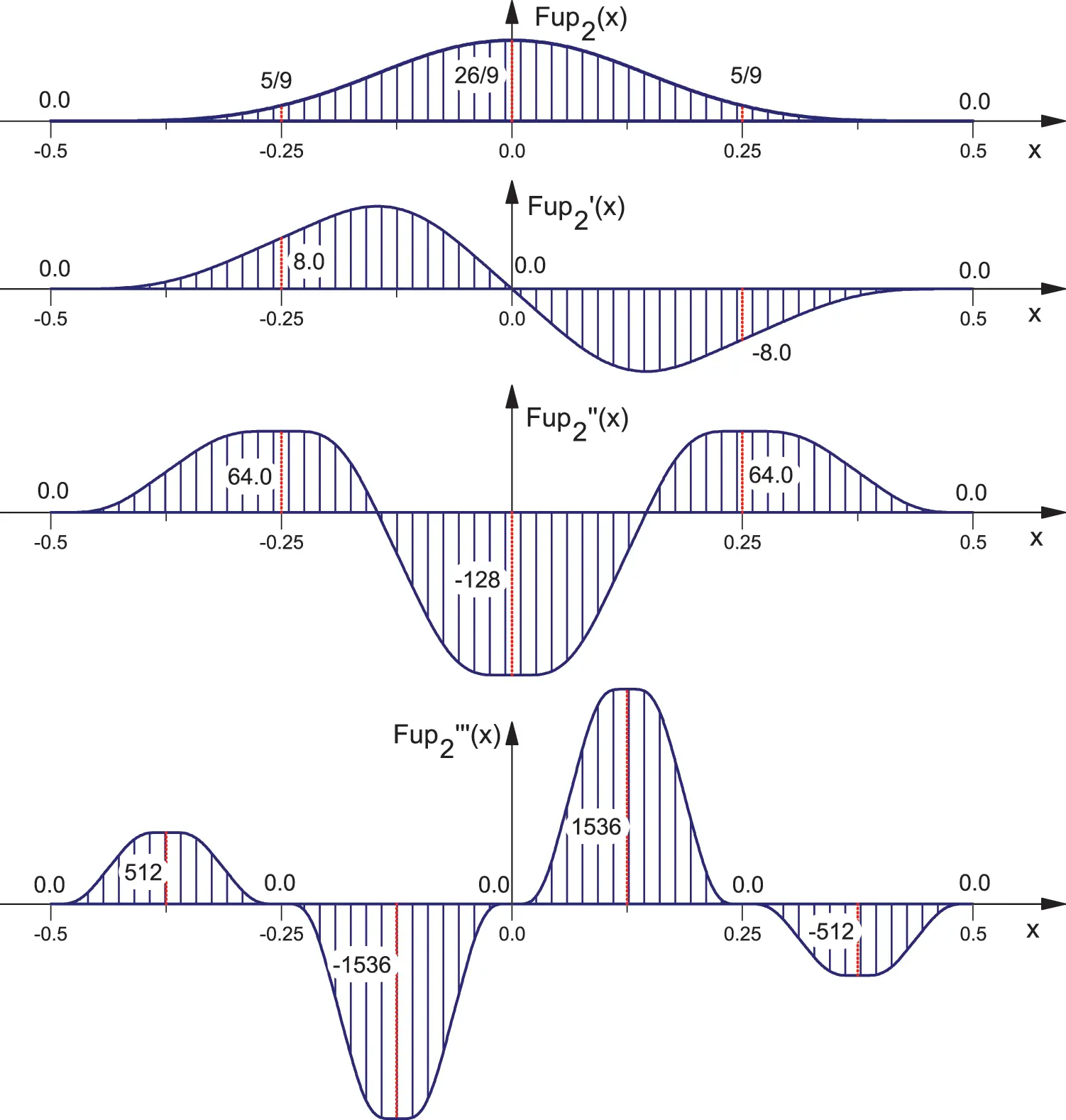Figure 1: Function Fup2(x) and it is first three derivatives

The integrals of the function Fupn(x) are also obtained by a linear combination of the integrals of the shifted functions up(x) using the coefficients from Eq. (11):

xFupn(x)dx=k=0Ckxup(x1k2n+n+22n+1)dx.(13)

3  ABFs of the Exponential Type: EFupn (x, ω)

The functions EFupn(x,ω) are finite functions of class C with compact support, and are the elements of linear vector space EUPn [12,27,28], and retain all the properties of their “maternal” basis function Eup(x,ω). The index ‘n’ denotes the largest degree of an exponential monomial that can be represented exactly in the form of a linear combination of mutually shifted functions EFupn(x,ω) on a characteristic segment of length Δxn=2n.

3.1 Generating the Fourier Transform of the Function EFupn(x, ω)

The Fourier transform of the atomic basis function EFupn(x,ω) is constructed by a similar procedure applied to the function Fupn(x) using the so-called “fragmentation process” of the FT as shown below.

The first from the ABF class of the exponential type EFupn(x,ω) for n=0 is precisely the “maternal” basis function Eup(x,ω), i.e.,

EFup0(x,ω)=Eup(x,ω).(14)

Thus, according to Eq. (14), the Fourier transform of the function EFup0(x,ω) is determined by the expression from :

F0(t)=j=1ω2sh(ω/2)sh(ω/2+it/2j)ω/2+it/2j.(15)

Writing Eq. (15) in an extended form, applying the basic trigonometric relations, and fragmenting the expression thus obtained (omitting the term ch(α/2)), after arranging the expression, we obtain the Fourier transform of the finite function EFup1(x,ω) of the form:

F1(t)=(ω2sh(ω/2)sh(ω/2+it/4)ω/2+it/4ω4sh(ω/4)sh(ω/4+it/4)ω/4+it/4)j=3ω2sh(ω/2)sh(ω/2+it/2j)ω/2+it/2j.(16)

The expression in parentheses from Eq. (16) represents the FT of the corresponding exponential spline, while the product from Eq. (16) is the FT of the function Eup(x,ω) from Eq. (15), condensed on the support [14,14] (see Fig. 2b). Continuing the presented procedure and generalizing it, we obtain the class of Fourier transforms of the exponential functions EFupn(x,ω) in the form: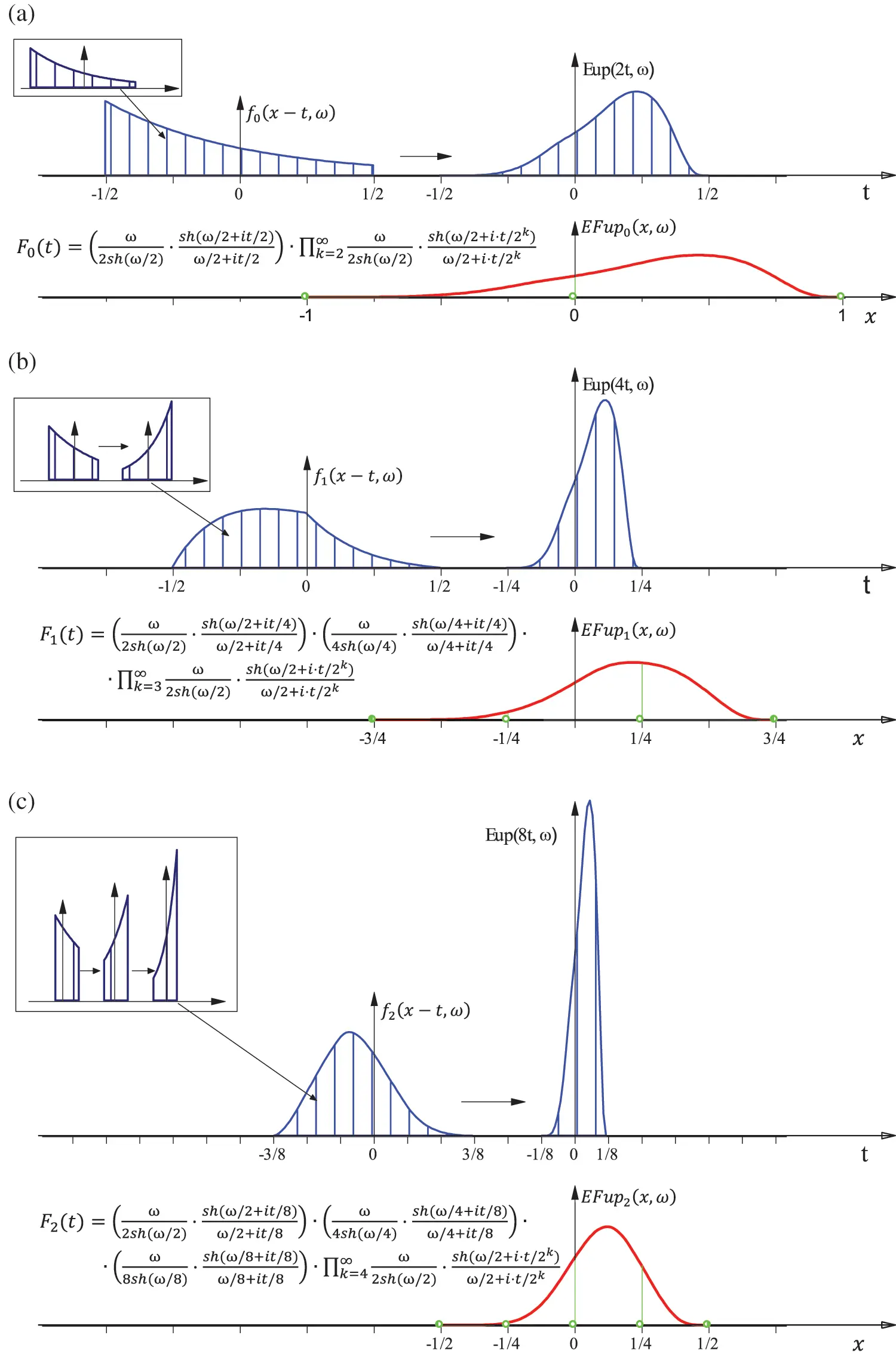Figure 2: Generating the exponential functions EFupn(x,ω) for: (a) n=0; (b) n=1; (c) n=2

Fn(t)=j=1n+1ω2jsh(ω/2j)sh(ω/2j+it/2n+1)ω/2j+it/2n+1k=n+2ω2sh(ω/2)sh(ω/2+it/2k)ω/2+it/2k.(17)

Thus, analogously to the ABF Fupn(x), according to Eq. (17), the function EFupn(x,ω) can be written using the convolution theorem in the following form:

EFupn(x,ω)=[φ00(x,ω)φ0n(x,ω)]2n+1Eup(2n+1x,ω),(18)

where

φ0j(x,ω)=2j1ωsh(ω/2nj+1)e2jωx,j=0,,n(19)

are the zero-degree exponential splines φ0j(x,ω) normalized to the support suppφ0j(x,ω)=[2(n+1),2(n+1)]. The convolution of splines φ0j(x,ω) in square brackets in Eq. (18) represents the corresponding exponential spline of nth degree fn(x,ω); thus, Eq. (18) can also be written in the form:

EFupn(x,ω)=fn(x,ω)2n+1Eup(2n+1x,ω).(20)

When the parameter ω weighs zero, the exponential ABF turns into an algebraic ABF, so Eqs. (17) and (20) become Eqs. (4) and (6), respectively.

Fig. 2 shows a graphical interpretation of Eq. (18) (or Eq. (20)), i.e., the procedure for generating the function EFupn(x,ω),n=0,1,2 using the convolution theorem. For example, the function EFup2(x,ω) represents the convolution of four functions normalized to the support [18,18]: three zero-degree exponential splines and the condensed Eup(x,ω) function, as shown in Fig. 2c, i.e., EFup2(x,ω)=ωeωx2sh(ω8) * ωe2ωxsh(ω4) * 2ωe4ωxsh(ω2) * 8Eup(8x,ω).

According to Eq. (18), the support of the function EFupn(x,ω) is an interval composed of (n+2) segments of length 2n. The characteristic points are the boundary points of the characteristic segment.The inverse Fourier transform, i.e., the function EFupn(x,ω), having satisfied the Paley–Wiener normalization condition, can be expressed in the form:

EFupn(x,ω)=12πeitxFn(t)dt.(21)

By developing Eq. (21) in the Fourier series, the “original” of the function EFupn(x,ω) can be determined at arbitrary points. However, as for the algebraic ABFs Fupn(x), the most favorable possibility of constructing the EFupn(x,ω) functions is in the form of a linear combination of shifted Eup(x,ω) functions, as shown below.

Fig. 3 shows the function EFup2(x,ω) for different values of the parameter ω. Similar to the “maternal” function Eup(x,ω), the function is tilted to the left for negative values of the parameter ω, while for positive ones it is tilted to the right. In the limitary case when ω0, the exponential function EFup2(x,ω) is identically equal to the algebraic function Fup2(x).Figure 3: Function EFup2(x,ω) for different values of the parameter 10ω10

3.2 Functional Differential Equation of the Function EFupn(x,ω)

Analogous to the algebraic ABF Fupn(x), the functional differential equation of the function EFupn(x,ω) is determined from the Fourier transform (17), which can also be written as follows:

Fn(t)=ω2n+1sh(ω/2n+1)sh(ω/2n+1+it/2n+1)ω/2n+1+it/2n+1k=2n+1ch(ω/2k+it/2n+2)ch(ω/2k)Fn(t2).(22)

If Eq. (22) is written in the form of exponential functions and multiplied by (ω+it), arranging the members of the left and right sides gives the functional differential equation of the function EFupn(x,ω) of the form:

EFupn(x,ω)ωEFupn(x,ω)=i=12n+1αiEFupn(2x+βi,ω),(23)

where the coefficients are

βi=±{j22sforn=2s(2j+1)22sforn=2s1;j=1,2,,2s(24)

and

αi=2ωeω1[Ain(ω)An+2in(ω)],(25)

where

Ain(ω)={0fori>neω/2nAi1n1(ω)+Ain1(ω)forin;A00(ω)=eω/2.(26)

In particular, when the value of the parameter ω=0, Eqs. (23)(26) become equivalent to Eq. (8).

3.3 The Values of the Function EFupn(x, ω) in Characteristic Points

The values of the functions EFupn(x,ω) at arbitrary discrete points x can be determined by Convolution (20) as a solution of the following integral:

EFupn(x,ω)=2n+1fn(xt,ω)Eup(2n+1t,ω)dt,(27)

where fn(x,ω) is the corresponding exponential spline defined as the result of the convolution of zero-degree exponential splines, i.e.,

fn(x,ω)=φ00(x,ω)φ0n(x,ω),(28)

while φ0j(x,ω) are determined by Eq. (19).

However, calculating the integral (27) at arbitrary points x is not a simple or numerically favorable procedure, and therefore solving the integral (27) is used only to determine the values of the basis functions EFupn(xk,ω) at the characteristic points xk.

Fig. 4 shows the graphical interpretation of the integral (27) for the basis function EFup2(x,ω) at the characteristic points xk=14,0,14.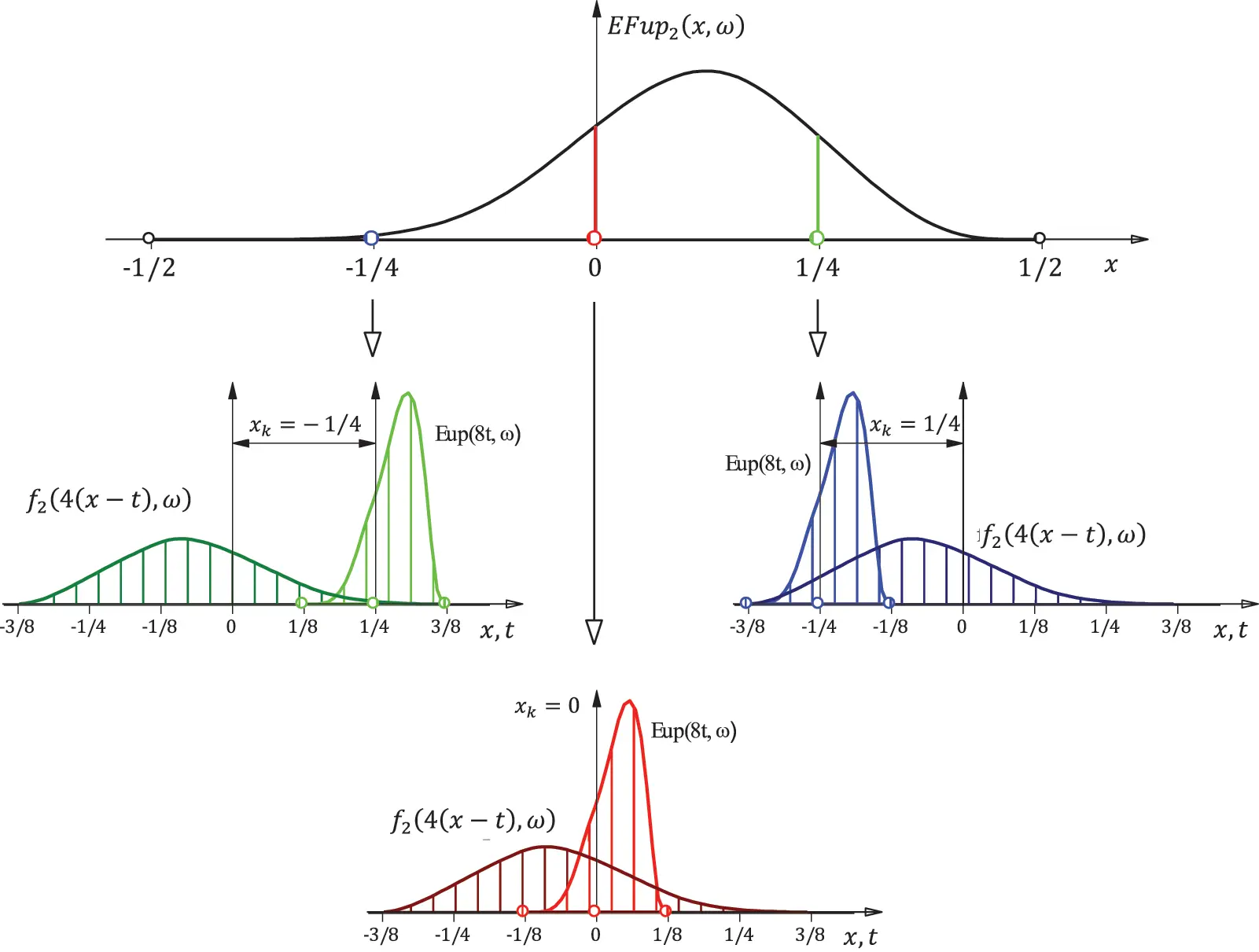Figure 4: The values of the function EFup2(x,ω) at the characteristic points xk=14,0,14 using the convolution theorem

For example, the value of the function EFup2(x,ω) at the point xk=1/4, according to Eq. (27), corresponds to the solution of the following integral:

EFup2(xk,ω)=81/8xk+3/8f2(xkt)Eup(8t,ω)dt,(29)

which, when written in exponential form and using the appropriate substitutions after arranging, has the final form:

EFup2(14,ω)=e3ω/4(e3ω/4+3eω/2+3eω/4+3)3(eω/4+1)(eω/2+eω/4+1)λ0(ω),(30)

where λ0(ω) is the value of the “maternal” function Eup(x,ω) at the local origin, i.e., the point x=0, see .

The values at other characteristic points of the function EFup2(x,ω) are determined by an analogous procedure:

EFup2(0,ω)=eω/4(3eω+6e3ω/4+8eω/2+6eω/4+3)3(eω/2+eω/4+1)λ0(ω),EFup2(14,ω)=e3ω/4(3e3ω/4+3eω/2+3eω/4+1)3(eω/4+1)(eω/2+eω/4+1)λ0(ω),(31)

or the values of the basis functions EFupn(x,ω) at the characteristic points in general.

As seen in Eqs. (30) and (31), the values of the functions EFupn(x,ω) at the characteristic points xk have a “final” inscription in the form of the product of the corresponding exponential function and the values of the function Eup(x,ω) at the point x=0, i.e., λ0(ω) given in .

3.4 EFupn(x, ω) as a Linear Combination of Shifted Eup(x, ω) Functions

The values of the basis functions EFupn(x,ω) at arbitrary points can be determined, among other methods, by developing Eq. (21) in the Fourier series. However, analogous to the algebraic ABF, the most favorable possibility of constructing the functions EFupn(x,ω) is in the form of a linear combination of mutually shifted Eup(x,ω) basis functions:

EFupn(x,ω)=k=0Ck(n)Eup(x1k2n+n+22n+1,ω),(32)

where Ck(n) are the coefficients of the linear combination.

The “zeroth” coefficient C0(n) is determined in  by the expression

C0(n)=i=1n(eω/2ni+1+1)i.(33)

The other coefficients of the linear combination Ck(n),k=1,,n+1 are unknown and are determined as described below.

For example, for the basis function EFup2(x,ω), the linear combination (32) has the following form (hereinafter, the functions Eup(x,ω) will be denoted by yω(x) for transparency):

EFup2(x,ω)=C0(2)yω(x12)+C1(2)yω(x34)+C2(2)yω(x1)+C3(2)yω(x54),(34)

or written in characteristic points:

EFup2(14)=C0(2)yω(34);EFup2(0)=C0(2)yω(12)+C1(2)yω(34);EFup2(14)=C0(2)yω(14)+C1(2)yω(12)+C2(2)yω(34);EFup2(12)=C0(2)yω(0)+C1(2)yω(14)+C2(2)yω(12)+C3(2)yω(34)=0,(35)

where the values of the basis function EFup2(x,ω) at the characteristic points are known and are calculated as shown in Section 3.3.

The expression for the “zeroth” coefficient follows directly from the first equation in Eq. (35):

C0(2)=EFup2(1/4)yω(3/4).(36)

By including the values from (30) and yω(3/4) from  in Eq. (36), we obtain

C0(2)=(eω/4+1)(eω/2+1)2,(37)

which corresponds to Eq. (33) for n=2.

The “first” coefficient of the linear combination (34) follows from the second equation in Eq. (35) in the form:

C1(2)=EFup2(0)C0(2)yω(1/2)yω(3/4).(38)

By including the coefficient C0(2) and the other required values, we obtain

C1(2)=eω/4(eω/4+1)(eω/2+1).(39)

The expression for the “third” coefficient follows from the third equation in Eq. (35), and so on. By generalizing the presented procedure, a general expression for the coefficients Ck(n) is obtained in the form of a recursive formula:

Ck(n)=EFupn(n+22n+1+k+12n,ω)i=1kCi1(n)Eup(1+k+2i2n,ω)Eup(1+2n,ω),(40)

where the coefficients C0(n) are determined by (33).

Thus, to determine the coefficients Ck(n),k=0,,n+1 of the linear combination (32), it is necessary to know the “zeroth” coefficient C0(n) and the values of the functions Eup(x,ω) and EFupn(x,ω) at the characteristic points xk.

In the limit when ω0, the coefficients Ck(n) for the development of the exponential functions EFupn(x,ω),n=1,,6 from Eq. (40) become the coefficients Ck(n) for the development of the algebraic basis functions Fupn(x) in the form of a linear combination of mutually shifted functions up(x) from Eq. (9).

Fig. 5 shows the function EFup2(x,ω) in the form of a linear combination of mutually shifted Eup(x,ω) basis functions.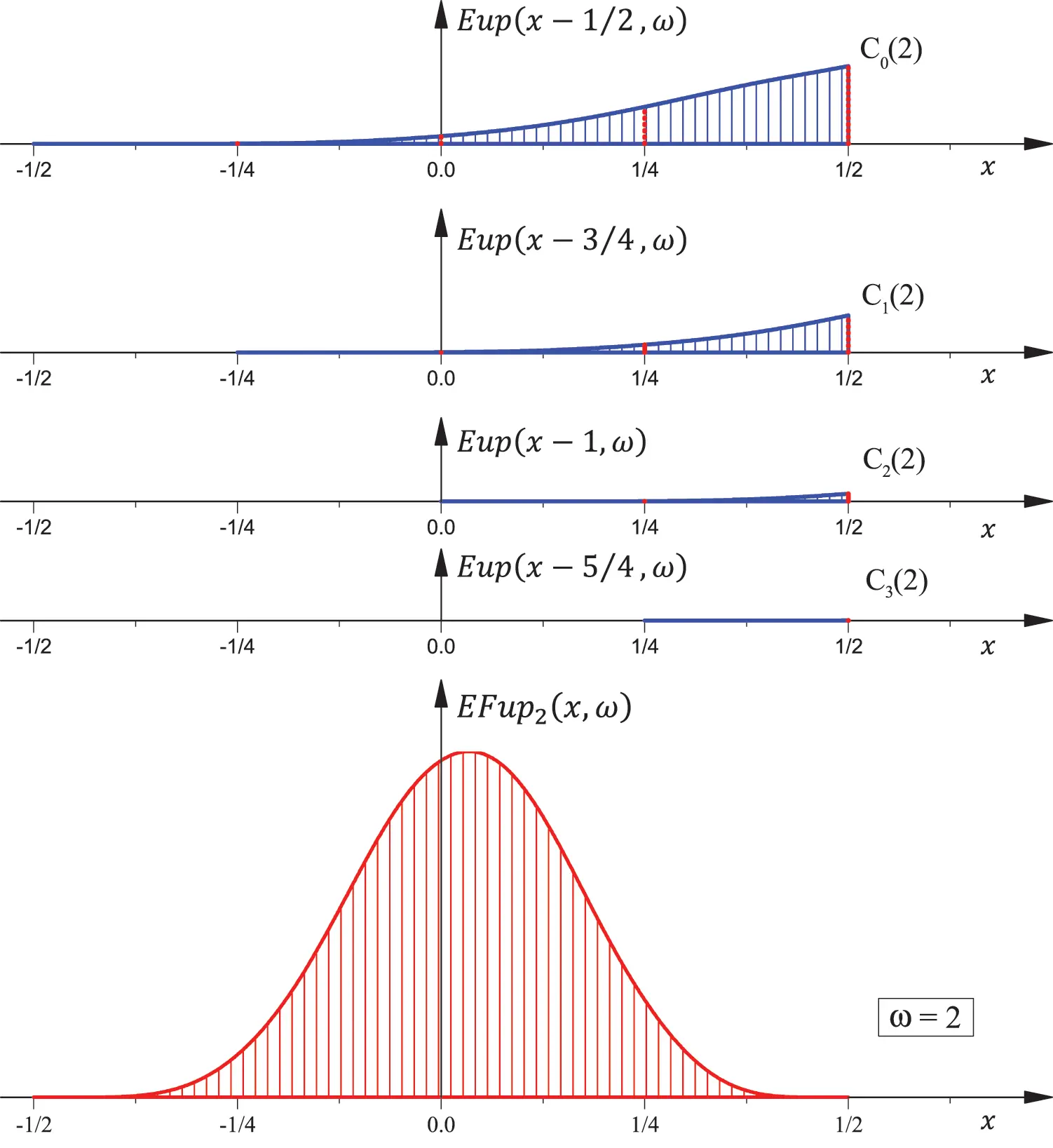Figure 5: Function EFup2(x,ω) as a linear combination of shifted Eup(x,ω) basis functions

3.5 The Derivatives and Integrals of the Function EFupn(x, ω)

The derivatives of the function EFupn(x,ω) are obtained by a linear combination of the derivatives of the shifted Eup(x,ω) functions using the coefficients specified in the previous section:

EFupn(m)(x,ω)=k=0Ck(n)Eup(m)(x1k2n+n+22n+1,ω).(41)

Fig. 6 shows the basis function EFup2(x,ω) and its first three derivatives for the value of the parameter ω=2.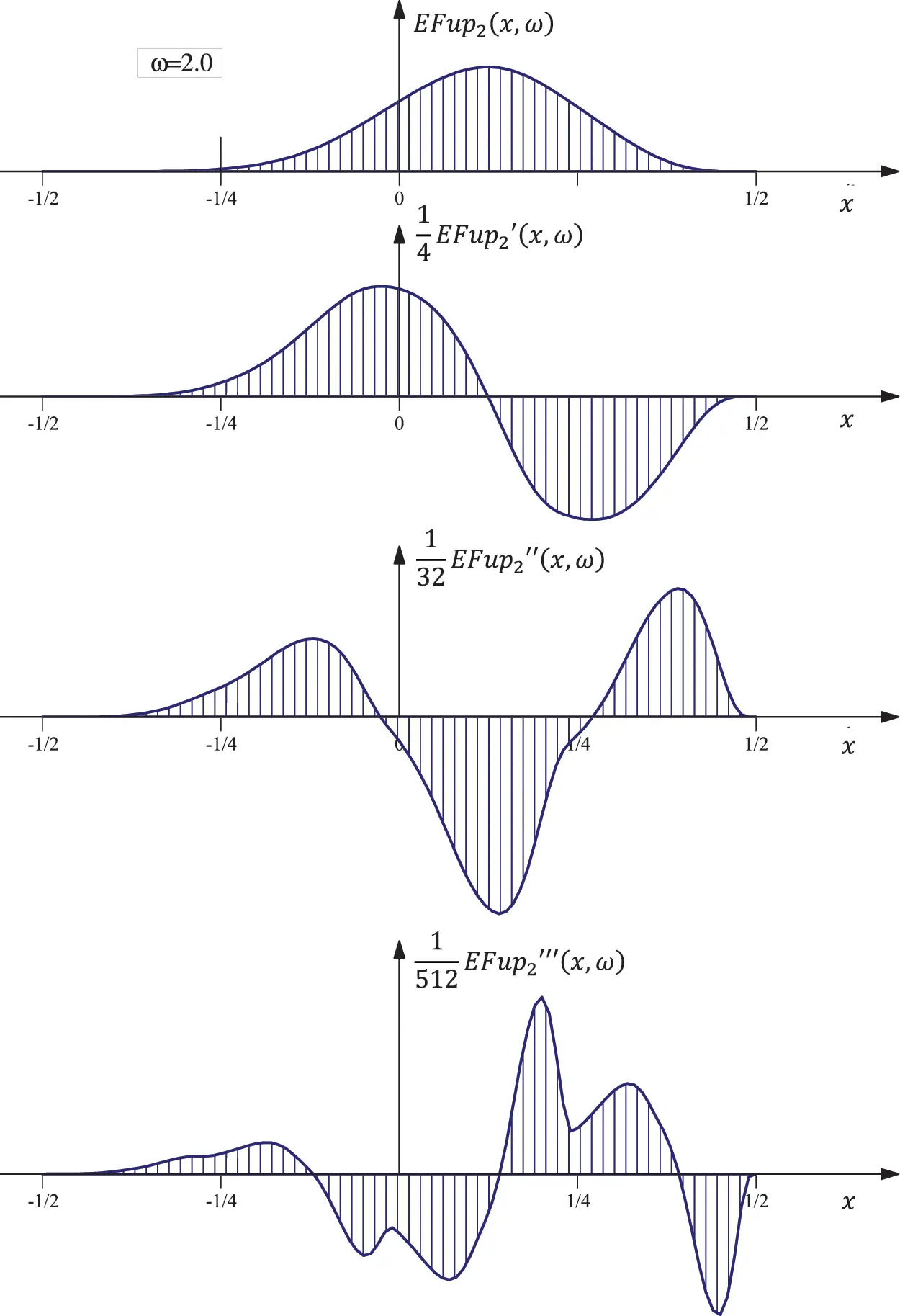Figure 6: Function EFup2(x,ω) and the first three derivatives

The integrals of the function EFupn(x,ω) are also obtained by a linear combination of the integrals of the shifted Eup(x,ω) functions:

xEFupn(x,ω)dx=k=0Ck(n)xEup(x1k2n+n+22n+1,ω)dx.(42)

3.6 The Connection between the Function EFupn(x, ω) and the Exponential Monomials

Similar to the function Eup(x,ω) , which is only a special case of the function EFupn(x,ω) for n=0, a connection between the functions EFupn(x,ω) and exponential monomials e2nωx can be established.

For a linear combination of the basis functions EFupn(x,ω),

φ(x)=k=Ck(n)EFupn(xk2n,ω),(43)

(offset from each other for the characteristic section Δxn=2n) to represent an exponential monomial of degree n, it is necessary and sufficient that by the action of the differential operator from [12,28] for a given nN

Ln=j=1n(d/dx2j1ω)(44)

on Eq. (43), the linear combination on the right is annuled.

For example, we show the calculation of the coefficients in the case of the basis function EFup2(x,ω). Fig. 7 shows the disposition of the basis functions EFup2(x,ω). Such an disposition of the basis functions accurately develops the exponential monomials up to and including the second degree, as well as the exponential polynomials formed by their combination. By the action of the operator from Eq. (44) on Eq. (43) for n=2, the following recursion is obtained: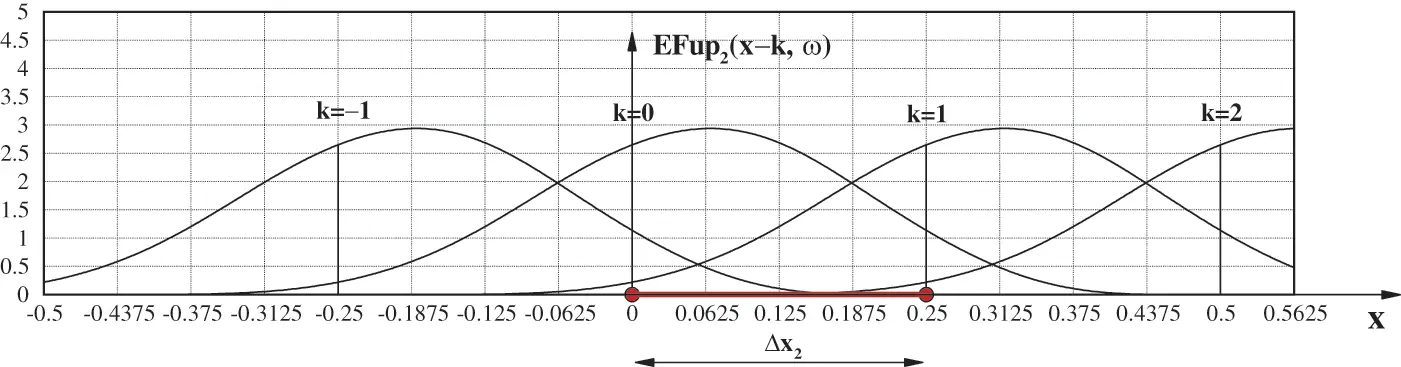Figure 7: Composition of the EFup2(x,ω) functions in a linear combination to obtain monomials φ(x)=eωx2n

a1Ck1(2)+a2Ck(2)+a3Ck+1(2)+a4Ck+2(2)=0,(45)

where

a1=64ω3e7ω/4/Na2=64ω3e3ω/4(e3ω/4+eω/2+1)/Na3=64ω3eω/4(e3ω/4+eω/4+1)/Na4=64ω3/NN=(eω/4+1)2(1eω/4)3(eω/2+1)

or, after reordering:

e7ω/4Ck1(2)+e3ω/4(e3ω/4+eω/2+1)Ck(2)+eω/4(e3ω/4+eω/4+1)Ck+1(2)+Ck+2(2)=0.(46)

By introducing the substitution Ck(2)=λk in Eq. (46), we obtain a characteristic equation whose roots are

λ0=eω/4;λ1=eω/2;λ2=eω.(47)

“Recompositioning” the roots (47) gives the general form of the coefficients Ck(n) for n=2:

Ck(2)=A0(2)e(ω/4)k+A1(2)e(ω/2)k+A2(2)eωk,(48)

or

Ck(2)=j=0nAj(2)eωk/2nj.(49)

The coefficients Aj(2) from Eq. (49) are calculated from the following system of equations:

Ck1(2)EFup2(1/4,ω)+Ck(2)EFup2(0,ω)+Ck+1(2)EFup2(1/4,ω)=eωkΔxCk(2)EFup2(1/4,ω)+Ck+1(2)EFup2(0,ω)+Ck+2(2)EFup2(1/4,ω)=eω(k+1)ΔxCk+1(2)EFup2(1/4,ω)+Ck+2(2)EFup2(0,ω)+Ck+3(2)EFup2(1/4,ω)=eω(k+2)Δx

For k=0 and Δx=1/4, we obtain

A0(2)=1eω/4EFup2(1/4,ω)+EFup2(0,ω)+eω/4EFup2(1/4,ω),A1(2)=0,A2(2)=0.

In general, the exponential monomial e2mωx,m=0,1,,n,nN on a segment of length 2n can be accurately represented by the linear combination of the (n+2)2n basis functions EFupn(x,ω) offset from each other by 2n in the form:

e2mωx=k=e2mωkΔxnAn(m)EFupn(xkΔxn,2nωΔxn);m=0,1,,n,(50)

where the coefficients An(m) (calculated from Eq. (49) for x=0) are of the following form:

An(m)=i=(n+2)2n1(n+2)2n1e2mωiΔxnEFupn(iΔxn,2nωΔxn).(51)

4  Practical Use of the Exponential ABF EFupn (x, ω)

For practical use we created the efupnM module to calculate the values of the functions EFupn(x,ω) and their derivatives at arbitrary points. The use of the software modules comes down to simply describing a function in a similar way to that of, for example, the trigonometric function sine: sin(ωx+φ) sine (omega, xpoint, fi). Fig. 8 shows a graphical interpretation of the variables that need to be specified when using the efupnM module.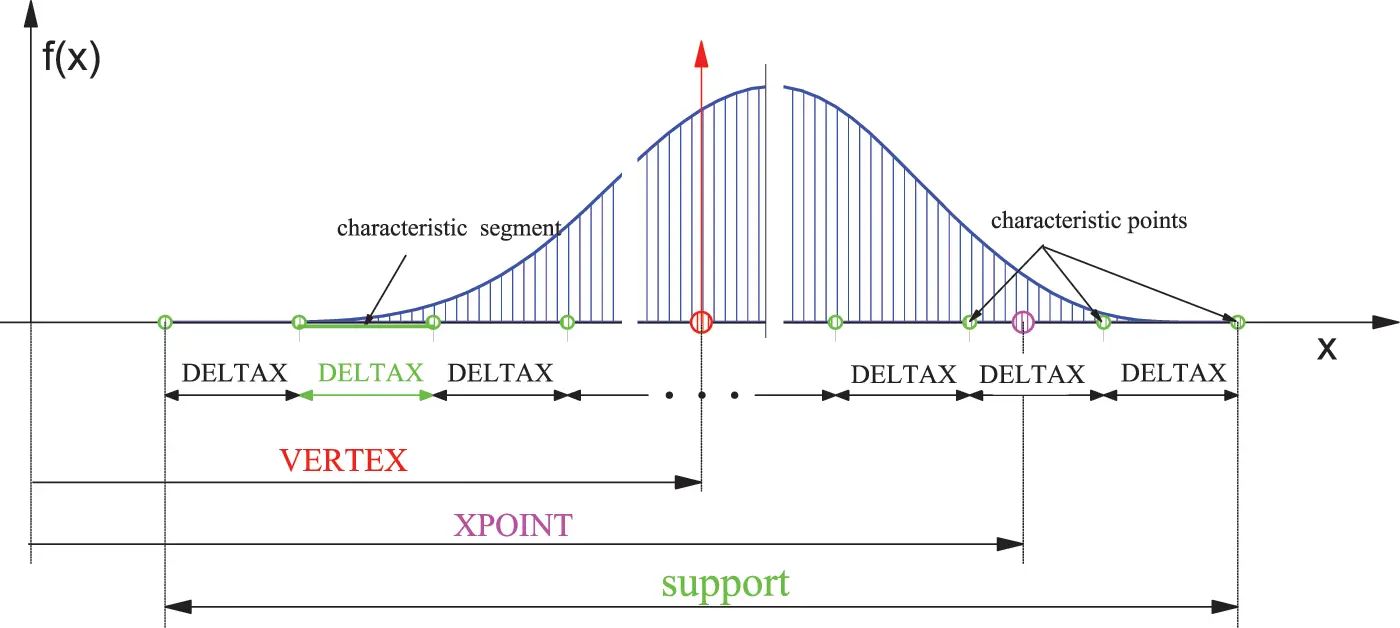Figure 8: Using the efupnM software module to calculate the values of the basis functions Fupn(x) and EFupn(x,ω)

dummy = efupnM (NFUP, OMEGA, VERTEX, DELTAX, XPOINT, KOD, NMAX),

where:

NFUP = n - the order of the function EFupn(x,ω);

OMEGA - frequency or tension parameter;

VERTEX - x-local coordinate system coordinates (located in the center of the support);

DELTAX - the real length of the characteristic segment;

XPOINT - the real x-coordinate of the arbitrary point at which the value of the function EFupn(x,ω) is sought;

KOD - the order of derivation of the function;

NMAX - accuracy parameter (depends on computer characteristics).

4.1 Determination of the Best Frequency in the Function Approximation

The basis functions of the exponential type, such as trigonometric functions, exponential splines, or ABFs of the exponential type, contain the parameter ω that provides them additional approximation properties. However, their application in numerical analysis is limited by the fact that the value of the parameter ω is, in most cases, unknown, and there is no universal criterion for choosing its value.

In this paper, the value of the parameter ω is determined using the least squares method by adopting the value of the parameter that gives the smallest deviation between a given function and its approximation at each characteristic segment of length ∆x. This method proved to be simple and efficient, and is shown in the example of the exponential function below.

Let there be given a function at the section AB¯=[0,1] in the form:

f(x)=e10(x1),x[0,1].(52)

Using the two characteristic segments of the length Δx=0.5 and the formation of the basis functions according to Fig. 7, the corresponding approximations are determined using the basis functions Fup2(x) and EFup2(x,ω).

As previously shown in Section 3.6, the linear combination of the basis functions EFupn(x,ω) identically approximates the exponential monomials (as well as their linear combination), i.e., the given function (52), for any number of basis functions or characteristic segments Δx in the region AB¯, as shown in Fig. 9. On the other hand, the approximation of the function (52) using the basis functions Fup2(x) shows a significant deviation from the given function on a small number of segments, as shown in Fig. 9.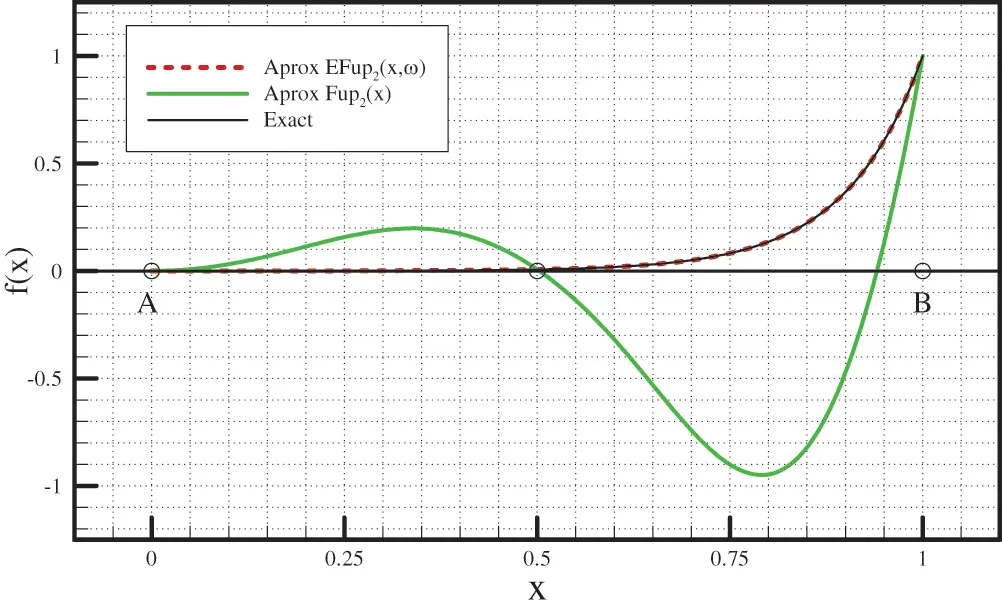Figure 9: Comparison of the given function (52) and approximations obtained using algebraic and exponential (frequency ω = 10) ABFs for n=2

Thus, the criterion for choosing the parameter’s value is in terms of least squares:

snk(ω)=k=1ns[f(x)f~(x)]2dx=min,(53)

where f(x) is a given function, f~(x) is an approximation of a given function, and ns is the number of characteristic segments Δx in the domain AB¯.

Fig. 10 shows the values of the least squares sum (53) for the approximations obtained by the values of the parameter ω in the interval [0,60] with a step Δω=0.1.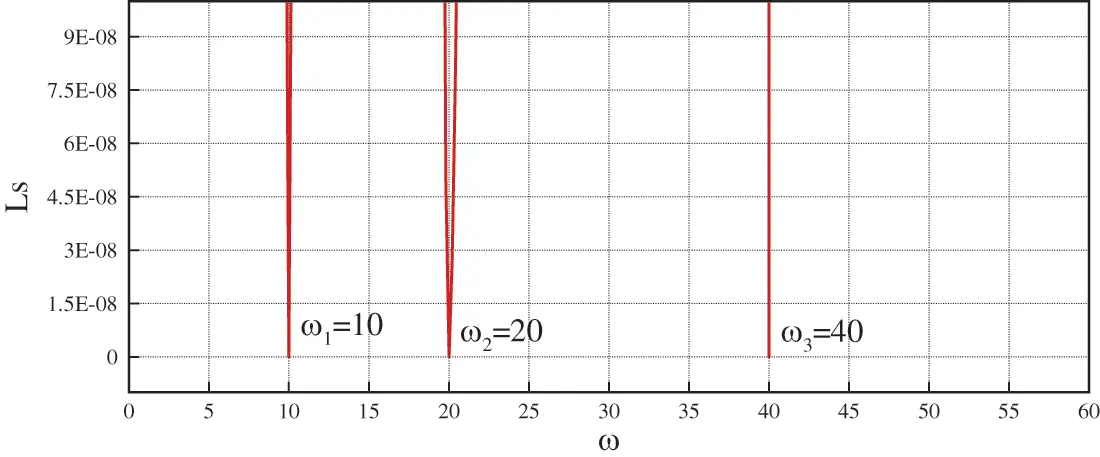Figure 10: Values of the least squares sum of the approximation obtained by the basis functions EFup2(x,ωi) for the different values of the parameter ω on the interval I=[0,60]

Since the frequency of the given function (52) is known and is ω=10, it is to be expected that, for the given value of ω, the least squares sum (53) for approximation by the exponential basis functions EFup2(x,ω) will be equal to zero; however, according to Section 3.6, for the values of the parameter ωi=2mω,m=0,1,2 also, which is confirmed in Fig. 10.

This confirms that the least squares method is a reliable, simple, and optimal choice of criteria for the determination of the value of the parameter ω.

4.2 Example 1: Approximation of a High-Degree Polynomial

An algebraic polynomial of degree 12 is approximated

f(x)=858x2(1x)10,x[0,1].(54)

Unlike the previous example where the value of the parameter ω followed from the function itself, here we have a “problem” of choosing the value of the parameter ω. The procedure for determining the value of the parameter ω is reduced to the simultaneous direct solution of the linear system of Eq. (43) using the point collocation method for different values of the parameter ω. Of all the numerical solutions thus obtained, the one that gives the minimum of the least squares function (53) for a given number ns of characteristic segments Δx is adopted.

Fig. 11 shows the values of the least squares sum of the approximation obtained by the EFup2(x,ω) basis functions on two characteristic segments for the values of the parameter ω on the interval I=[30,10] with the step Δω=0.1.Figure 11: Values of the least squares sum of the approximation obtained by the basis functions EFup2(x,ωi) for the values of the parameter ω on the interval I=[30,10]

The minimum value of the least squares sum for two characteristic segments was obtained for the approximation using the exponential basis functions EFup2(x,ω), when the value of the parameter ω=16.5, and is Ls=0.113453941003, while the value of the least squares sum of the approximation obtained by the algebraic basis functions Fup2(x), i.e., when the value of the parameter ω=0.0, for the same number of sections is 2.62204156514.

Fig. 12 shows a comparison of the given function (54) with the approximations obtained by the algebraic basis functions Fup2(x) and the exponential basis functions EFup2(x,ω) for four different segment lengths Δx=1/ns, where ns=2,4,8,16.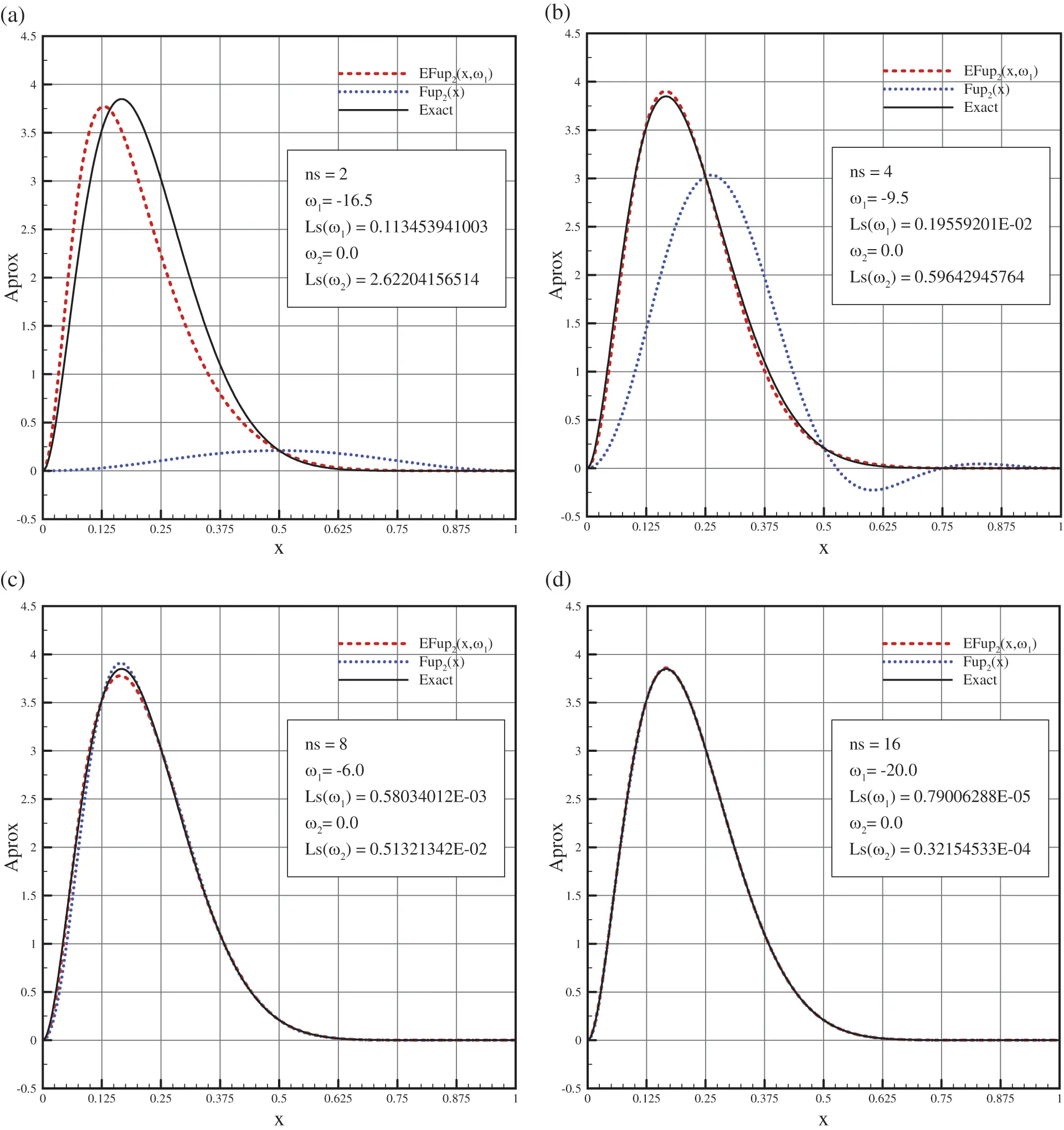Figure 12: Comparison of the approximations with a given function (54) using the algebraic and exponential ABFs for: (a) ns=2, (b) ns=4, (c) ns=8, and (d) ns=16

In Figs. 12a and 12b, it can be seen that for a small number of characteristic segments, the approximation by the exponential basis functions EFup2(x,ω) gives a significantly better approximation to the given function (54) than the approximation obtained by the algebraic functions Fup2(x), while as the number of segments (ns) increases, this difference in approximations decreases, as shown in Figs. 12c and 12d.

In order to draw a conclusion regarding the character of the convergence of the mentioned numerical approximations to a given function, it is necessary to perform a calculation by increasing the number of segments to a certain desired accuracy of the results. From Fig. 12, it can be seen that the best approximation is achieved using the exponential basis functions with different parameterω values depending on the number of segments in the area (ns). Fig. 13 shows the values of the parameter ω obtained by the least squares method in relation to the number of characteristic segments in the area. It can be seen that the value of the parameter ω is sensitive to discretization of the domain only for a small number of sections up to ns=16, while when the number of sections is greater than 16, the parameter ω has a constant value of 19.0. Therefore, the convergence diagram of the numerical solution for the exponential basis functions EFup2(x,ω) is obtained using the values ω=19.0.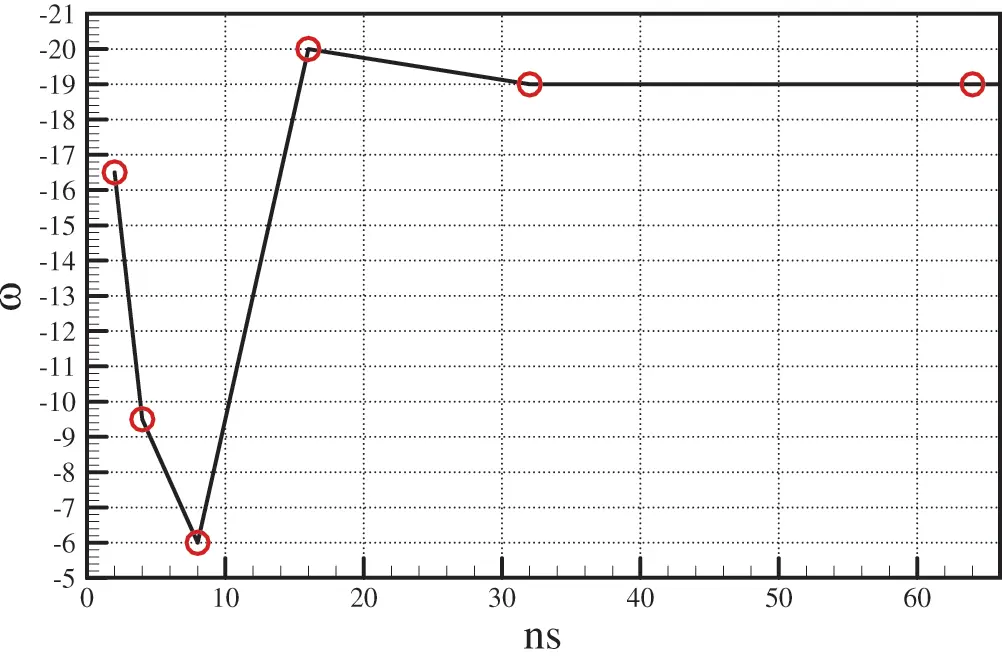Figure 13: Dependence of the parameter’s ω value on the number of characteristic segments in the approximation of the given function (54) using the EFup2(x,ω) basis functions

The diagrams in Fig. 14 show, on a logarithmic scale, the relationship between the error expressed over the L2-norm and the segment length Δx for the approximations obtained by the basis functions Fup2(x) and EFup2(x,ω). It can be observed that the approximation obtained by the exponential ABFs achieves greater accuracy compared to the approximation obtained by the algebraic ABFs. Both diagrams show that the expected convergence rate is achieved, which, for the problem of the approximation of a given function, is p=n+1.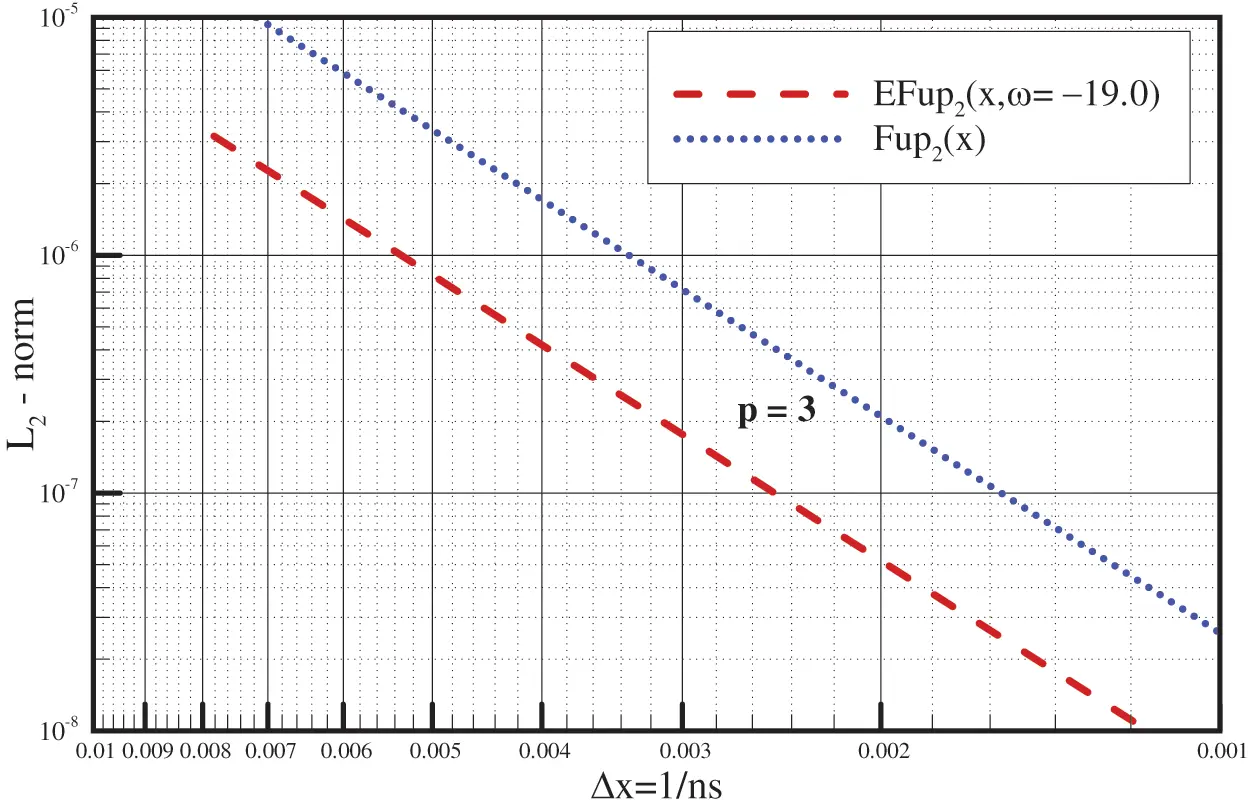Figure 14: Convergence diagrams of the accuracy of the numerical approximations obtained by the Fup2(x) and EFup2(x,ω) basis functions

4.3 Example 2: Approximation of a Sudden Jump Function

The following function on the interval [0,1] is analyzed:

f(x)=TANH((x0.5)/0.02).(55)

Fig. 15 shows a comparison of the given function (55) with the approximations obtained by the algebraic basis functions Fup2(x) and the exponential basis functions EFup2(x,ω) for the segments of the lengths Δx=1/ns where ns=2,4,8,16. It can be observed that exponential ABFs better describe the given function near the jump, while in the parts of the domain where the given function has a constant value, the approximation obtained by EFup2(x,ω) shows higher oscillations compared to the approximation obtained by Fup2(x) function. Fig. 15 also shows that, for this example of the function with a sudden jump, the exponential basis functions EFup2(x,ω) achieve a better approximation than the algebraic Fup2(x) basis functions for a smaller number of segments in the domain, while for a larger number of segments, the accuracy of the approximation equates that obtained using the functions Fup2(x).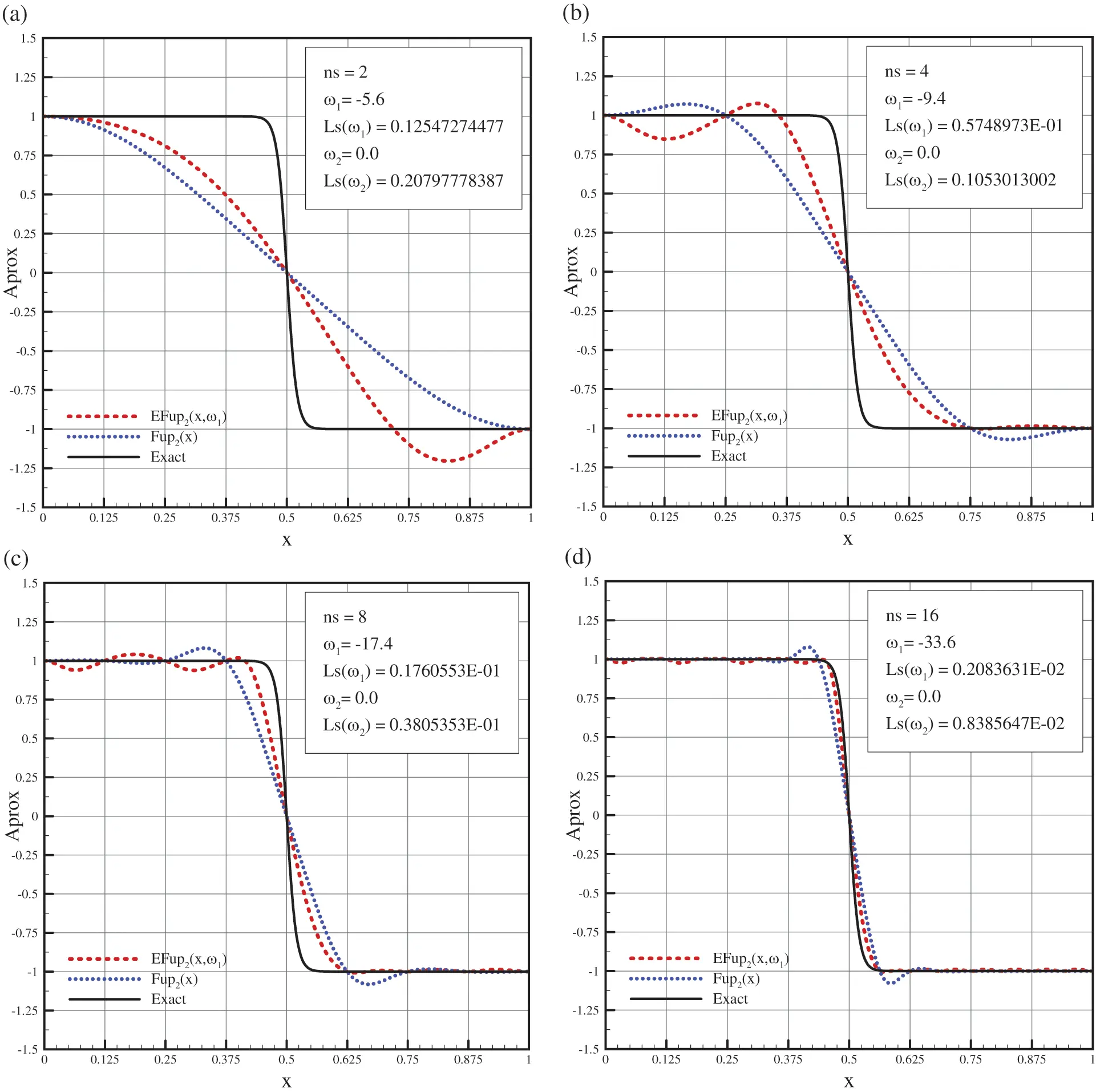Figure 15: Comparison of the approximations with a given function (55) using the algebraic and exponential basis functions for: (a) ns=2, (b) ns=4, (c) ns=8, and (d) ns=16

4.4 Example 3: Solving the Differential Equation of Conduction

Let a given differential equation of conduction with corresponding boundary conditions be

d2udx2+ωdudx=0;u(0)=0;u(1)=1.(56)

with known exact solution of the form

u(x)=eωx1eω1.(57)

Fig. 16 shows the dependence of the solution of Eq. (56) on the parameter ω, and how, for high values of ω, the solution function shifts to the right boundary.Figure 16: Dependence of the exact solution of the conduction problem (56) on the frequency ω

Fig. 17 compares the exact solution (57) of the conduction problem (56) with the solutions obtained using the basis functions Fup2(x) and EFup2(x,ω) with the point collocation method for the characteristic segment Δx=0.25, i.e., with a total of seven basis functions on the domain.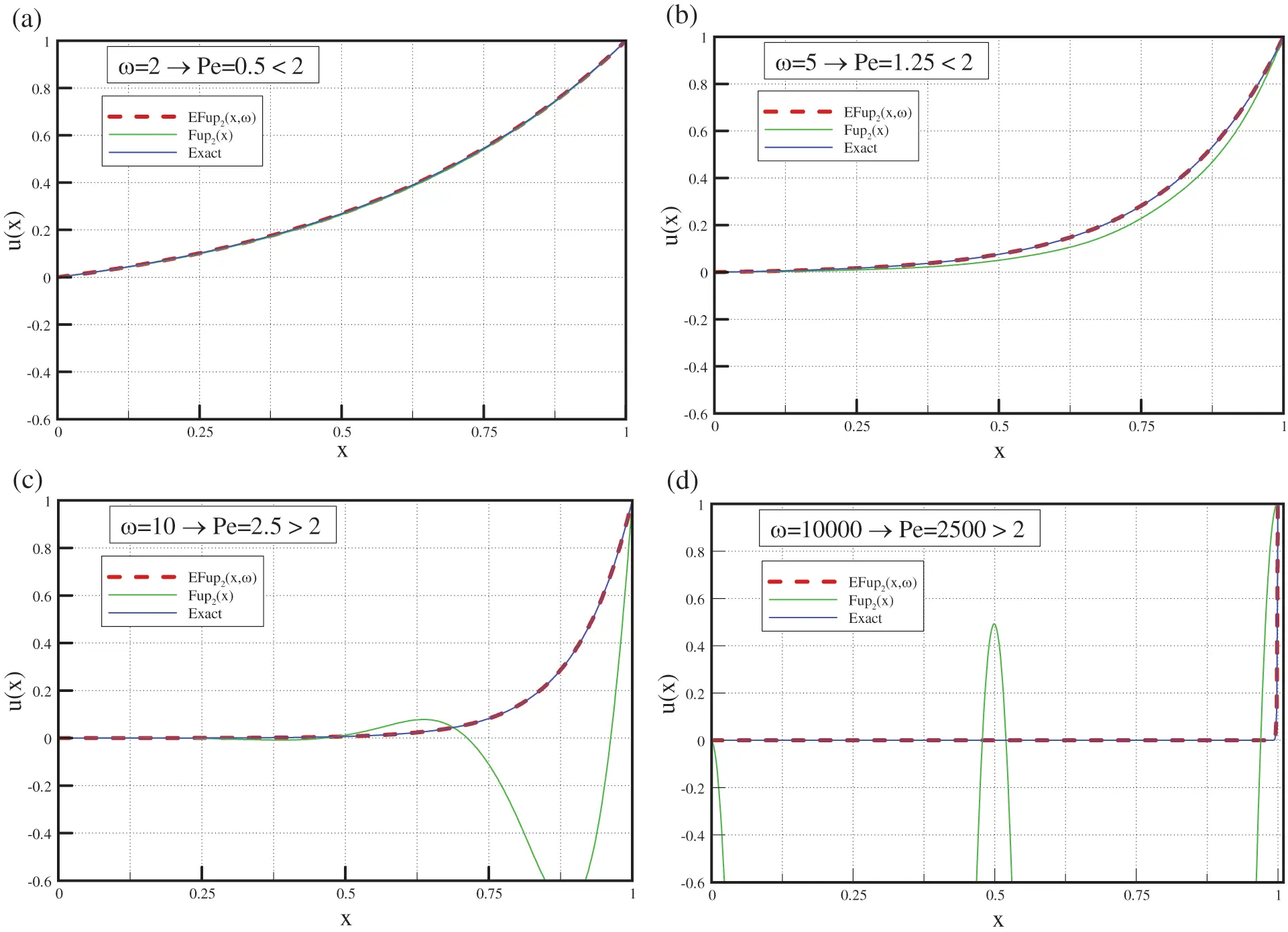Figure 17: Comparison of the numerical solutions of Eq. (56) obtained by the Fup2(x) and EFup2(x,ω) basis functions with the exact solution

Approximation using the basis functions of the algebraic type is limited by the Peclet number Pe=Δxω<2 because, at high values of the Pe, there is a numerical error and oscillation in the approximate solution. For the atomic basis functions of the exponential type there is no such a restriction.

In Fig. 17a for ω=2 and Pe=0.52, the solutions coincide with the exact solution. In Fig. 17b for ω=5 and Pe=1.25<2, the solution obtained with EFup2(x,ω) fully corresponds to the correct solution, while the solution obtained with Fup2(x) shows a deviation from the exact one, but still does not oscillate. In Fig. 17c for ω=10 and Pe=2.5>2, the solution obtained with EFup2(x,ω) still fully corresponds to the exact solution, while the solution obtained with Fup2(x) begins to oscillate significantly around the exact solutions. In Fig. 17d, for ω=10000 and Pe=25002, the solution obtained with EFup2(x,ω) corresponds to the exact solution, while the solution obtained with Fup2(x) satisfies the boundary conditions and the differential equation at the collocation points but is completely unusable.

5  Conclusion

The current knowledge regarding algebraic atomic basis functions Fupn(x) is synthesized in the paper. Their basic properties are described, and the expressions for the necessary mathematical operations are presented in a simpler, more understandable, and user-friendly way. Very little was known about the ABFs of the exponential type, and they were developed only at the basic level in [12,27,28]. In this paper, the basic properties of the functions EFupn(x,ω) are shown using the same approach as that for the ABFs of the algebraic type. The expressions for calculating the values of the functions and the desired number of derivatives at arbitrary points of the basis function support and, most importantly, the rules (elements) for their practical use are derived. The EFupnM software module for the practical application of these functions is also shown.

In the examples of the approximations of given functions, namely, a high-degree algebraic polynomial representing an asymmetric function and functions with a sudden jump, the exponential basis functions EFupn(x,ω) show better properties compared to the basis functions of the algebraic type Fupn(x). This is especially evident in approximations that use a smaller number of basis functions. As the number of basis functions in the region increases, the approximation properties EFupn(x,ω) of the functions are equated with the properties of the functions Fupn(x). The advantage of the EFupn(x,ω) function comes to expression especially when solving a differential equation of conduction that has an exact solution in the form of an exponential-type function. The exponential basis functions give a better approximate solution of high accuracy with the absence of the oscillations of the numerical solution.

Algebraic atomic basis functions have been used for many years to solve various numerical problems, and their advantage over other basis functions has become unquestionable. The ABFs of the exponential type show even better approximation properties, as demonstrated in this paper. The only question that still remains open is the choice of the value of the tension parameter ω. As with exponential splines, this complex issue requires further research both in one-dimensional problems and in the higher dimensions of space. In this paper, for the parameter selection criterion, we used the least squares sum, which proved to be simple and reliable. However, a disadvantage was the additional CPU time required to simultaneously solve the system of equations for the purpose of obtaining the approximations for different values of the parameter ω. This could be reduced by reducing the search interval of the parameter values according to the properties of a given numerical problem, i.e., whether it is an approximation of a given function or solving a differential equation.

Our further research should include an improvement of the procedure for finding the optimal value of the tension parameter. The natural sequence of development and application of the ABF of the exponential type leads to 2D and 3D numerical analysis. The advantage of ABF of the exponential type can be suitable for the application of adaptive procedures in the problems of computational mechanics.

Funding Statement: This research is partially supported through Project KK.01.1.1.02.0027, a project co-financed by the Croatian Government and the European Union through the European Regional Development Fund-the Competitiveness and Cohesion Operational Programme.

Conflicts of Interest: The authors declare that they have no conflicts of interest to report regarding the present study.

## References

1. Zienkiewicz, O. C., Taylor, R. L. (2002). The finite element method. Oxford: Butterworth-Heinemann.
2. Sauter, S. A., Schwab, C. (2011). Boundary element methods. Berlin, Heidelberg: Springer.
3. Tavarez, F. A., & Plesha, M. E. (2007). Discrete element method for modelling solid and particulate materials. International Journal for Numerical Methods in Engineering, 70(4), 379-404. [Google Scholar] [CrossRef]
4. Belytschko, T., Krongauz, Y., Organ, D., Fleming, M. A., & Krysl, P. (1996). Meshless methods: An overview and recent developments. Computer Methods in Applied Mechanics and Engineering, 139(1–2), 3-47. [Google Scholar] [CrossRef]
5. Liu, G. R., Gu, Y. T. (2005). An introduction to meshfree methods and their programming. Dordrecht: Springer.
6. Fries, T. P., Matthies, H. G. (2004). Classification and overview of meshfree methods. Brunswick: Institute of Scientic Computing Technical University Braunschweig.
7. Hölling, K., Hörner, J. (2013). Approximation and Modeling with B-splines. Philadelphia: Siam.
8. Hughes, T., Cottrell, J., & Bazilevs, Y. (2005). Isogeometric analysis: CAD, finite elements, nurbs, exact geometry and mesh refinement. Computer Methods in Applied Mechanics and Engineering, 194(39), 4135-4195. [Google Scholar] [CrossRef]
9. Cottrell, J. A., Hughes, T. J. R., Bazilevs, Y. (2009). Isogeometric analysis toward intergration of CAD and FEA. Wiley.
10. Rvachev, V. L., & Rvachev, V. A. (1971). On a finite function. Doklady Akademii Nauk Ukrainian SSR, A(6), 705-707. [Google Scholar]
11. Rvachev, V. L., Rvachev, V. A. (1979). Non-classical methods for approximate solution of boundary-value problems. Kiev: Naukova dumka.
12. Gotovac, B. (1986). Numerical modelling of engineering problems by smooth finite functions (Ph.D. Thesis). University of Split, Split (in Croatian).
13. Kravchenko, V. F. (2003). Lectures on the theory of atomic functions and their some applications. Moscow: Radiotechnika.
14. Beylkin, G., & Keiser, J. M. (1997). On the adaptive numerical solution of nonlinear partial differential equations in wavelet bases. Journal of Computational Physics, 132(2), 233-259. [Google Scholar] [CrossRef]
15. Gotovac, B., & Kozulić, V. (1999). On a selection of basis functions in numerical analyses of engineering problems. International Journal for Engineering Modelling, 12(1–4), 25-41. [Google Scholar]
16. Kolodiazhny, V. M., & Rvachev, V. A. (2007). Atomic functions: Generalization to the multivariable case and promising applications. Cybernetics and Systems Analysis, 43(6), 893-911. [Google Scholar] [CrossRef]
17. Kravchenko, V. F., Basarab, M. A., & Perez-Meana, H. (2001). Spectral properties of atomic functions used in digital signal processing. Journal of Communications Technology and Electronics, 46, 494-511. [Google Scholar]
18. Gotovac, B., & Kozulić, V. (2002). Numerical solving of initial-value problems by Rbf basis functions. Structural Engineering and Mechanics, 14(3), 263-285. [Google Scholar] [CrossRef]
19. Kozulić, V., & Gotovac, B. (2000). Numerical analyses of 2D problems using Fupn(x,y) basis functions. International Journal for Engineering Modelling, 13(1–2), 7-18. [Google Scholar]
20. Gotovac, H., Kozulic, V., & Gotovac, B. (2010). Space-time adaptive fup multi-resolution approach for boundary-initial value problems. Computers, Materials & Continua, 15(3), 173-198. [Google Scholar] [CrossRef]
21. Kozulic, V., & Gotovac, B. (2011). Elasto-plastic analysis of structural problems using atomic basis functions. Computer Modeling in Engineering & Sciences, 80(4), 251-274. [Google Scholar] [CrossRef]
22. Gotovac, H., Andricevic, R., Gotovac, B., Kozulic, V., & Vranjes, M. (2003). An improved collocation method for solving the Henry problem. Journal of Contaminant Hydrology, 64(1), 129-149. [Google Scholar] [CrossRef]
23. Gotovac, H., Cvetkovic, V., & Andricevic, R. (2009). Adaptive Fup multi-resolution approach to flow and advective transport in highly heterogeneous porous media: Methodology, accuracy and convergence. Advances in Water Resources, 32(6), 885-905. [Google Scholar] [CrossRef]
24. Kamber, G., Gotovac, H., Kozulić, V., Malenica, L., & Gotovac, B. (2020). Adaptive numerical modeling using the hierarchical Fup basis functions and control volume isogeometric analysis. International Journal for Numerical Methods in Fluids, 92(10), 1437-1461. [Google Scholar] [CrossRef]
25. Kravchenko, V. F., Kravchenko, O. V., Konovalov, Y. Y., & Budunova, K. A. (2020). Atomic functions theory: History and modern results: Dedicated to the pioneer of atomic functions theory V. L. Rvachev invited paper. IEEE Ukrainian Microwave Week, 43, 619-623. [Google Scholar] [CrossRef]
26. Kravchenko, V. F., Kravchenko, O. V., Pustovoit, V. I., Churikov, D. V., Volosyuk, V. K. et al. (2016). Atomic functions theory: 45 years behind. 9th International Kharkiv Symposium on Physics and Engineering of Microwaves, Millimeter and Submillimeter Waves (MSMW), pp. 1–4. DOI 10.1109/MSMW.2016.7538216.
27. Brajčić Kurbaša, N. (2016). Exponential atomic basis functions: Development and application (Ph.D. Thesis). University of Split, Split (in Croatian).
28. Brajčić Kurbaša, N., Gotovac, B., & Kozulić, V. (2016). Atomic exponential basis function Eup(x,ω)-development and application. Computer Modeling in Engineering & Sciences, 111(6), 493-530. [Google Scholar] [CrossRef]

Kurbaša, N. B., Gotovac, B., Kozulić, V. (2023). The Class of Atomic Exponential Basis Functions EFupn(x,ω)-Development and Application. CMES-Computer Modeling in Engineering & Sciences, 135(1), 65–90.This work is licensed under a Creative Commons Attribution 4.0 International License , which permits unrestricted use, distribution, and reproduction in any medium, provided the original work is properly cited.

View

Like

## Related articles

• H. Azoui, D. Bahloul, N. Soltani
• T. Chekifi, B. Dennai, R. Khelfaoui
• N. Soltani, S. Rahal
• A. Abbassi, N. Kechiche, H. Ben...
• A.Kurenkov, A.Thess, H.Babovsky...